Question

# 4. Draw the magnitude characteristic of the Bode plot of the following transfer function: 10s H...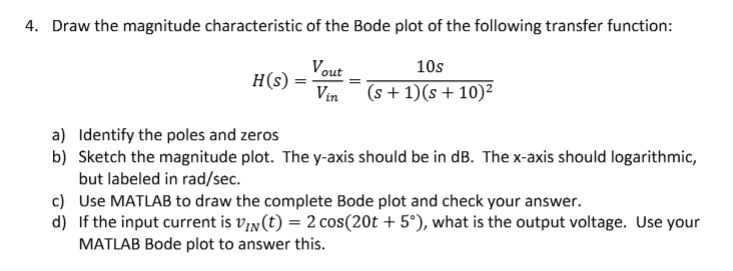4. Draw the magnitude characteristic of the Bode plot of the following transfer function: 10s H (s) =-= (s+1)(s+102 L7n Identify the poles and zeros Sketch the magnitude plot. The y-axis should be in dB. The x-axis should logarithmic, but labeled in rad/sec Use MATLAB to draw the complete Bode plot and check your answer. If the input current is un(t) = 2 cos(20t + 5°), what is the output voltage. Use your MATLAB Bode plot to answer this a) b) c) d)

We need at least 10 more requests to produce the answer.

0 / 10 have requested this problem solution

The more requests, the faster the answer.

All students who have requested the answer will be notified once they are available.

#### Earn Coins

Coins can be redeemed for fabulous gifts.

Similar Homework Help Questions
• ### 5. Draw the magnitude characteristic of the Bode plot of the following transfer function: H(s)10(10s +11...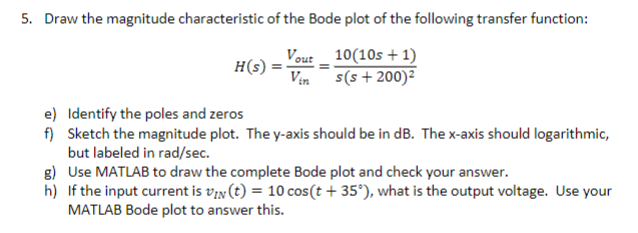5. Draw the magnitude characteristic of the Bode plot of the following transfer function: H(s)10(10s +11 (S + 200)2 Identify the poles and zeros Sketch the magnitude plot. The y-axis should be in dB. The x-axis should logarithmic, but labeled in rad/sec e) f) g) Use MATLAB to draw the complete Bode plot and check your answer. h) If the input current is VIN(t)-10 cos(t+35"),what is the output voltage. Use your MATLAB Bode plot to answer this.

• ### please answer all questions and show all steps 3. Draw the magnitude characteristic of the Bode...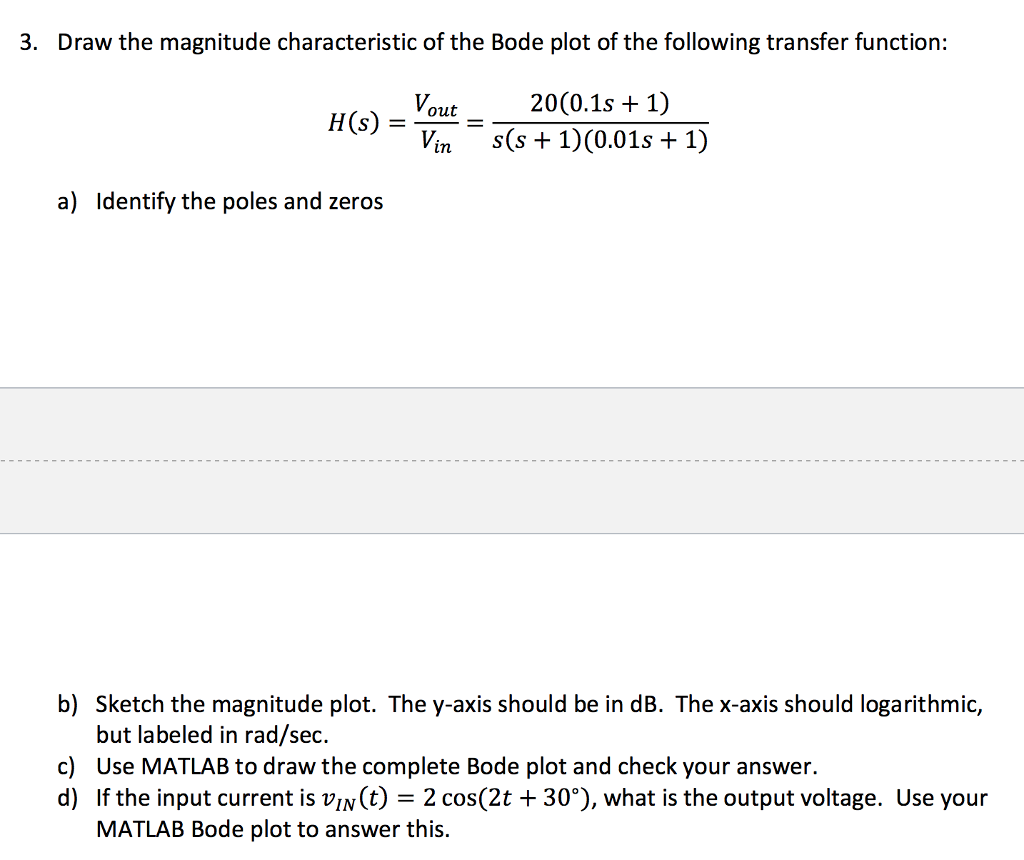please answer all questions and show all steps 3. Draw the magnitude characteristic of the Bode plot of the following transfer function: Hsout20(0.1s + 1 Vin S(s1)(0.01s +1) a) ldentify the poles and zeros b) Sketch the magnitude plot. The y-axis should be in dB. The x-axis should logarithmic, but labeled in rad/sec. Use MATLAB to draw the complete Bode plot and check your answer. If the input current is uln(t)-2 cos(2t + 30%), what is the output voltage. Use...

• ### Hw 10 Problem 2 2. Draw the magnitude characteristic of the Bode plot of the following...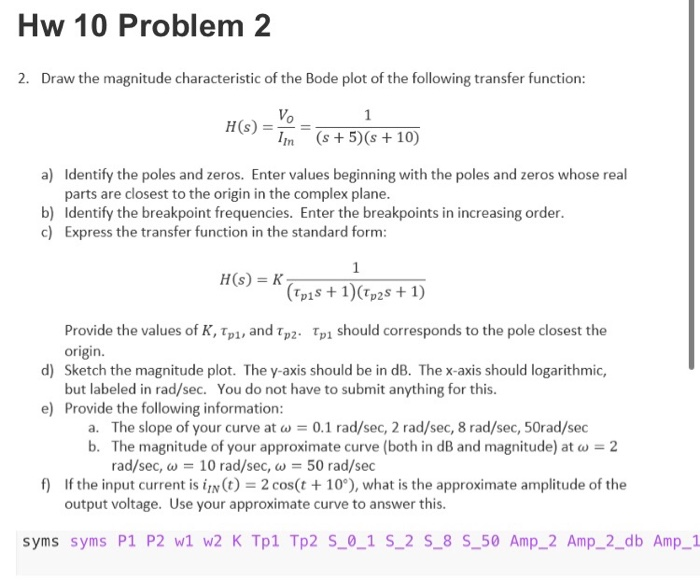Hw 10 Problem 2 2. Draw the magnitude characteristic of the Bode plot of the following transfer function: HG) = (s +5)(s +10) 5 + 5 + 10) a) Identify the poles and zeros. Enter values beginning with the poles and zeros whose real parts are closest to the origin in the complex plane. b) Identify the breakpoint frequencies. Enter the breakpoints in increasing order. c) Express the transfer function in the standard form: H(S) = (Tpis + 1)(Tp25 +...

• ### Chapter 12, Problem 12.21 (Multistep) Part 1 Correct. Sketch the magnitude characteristic of the Bode plot...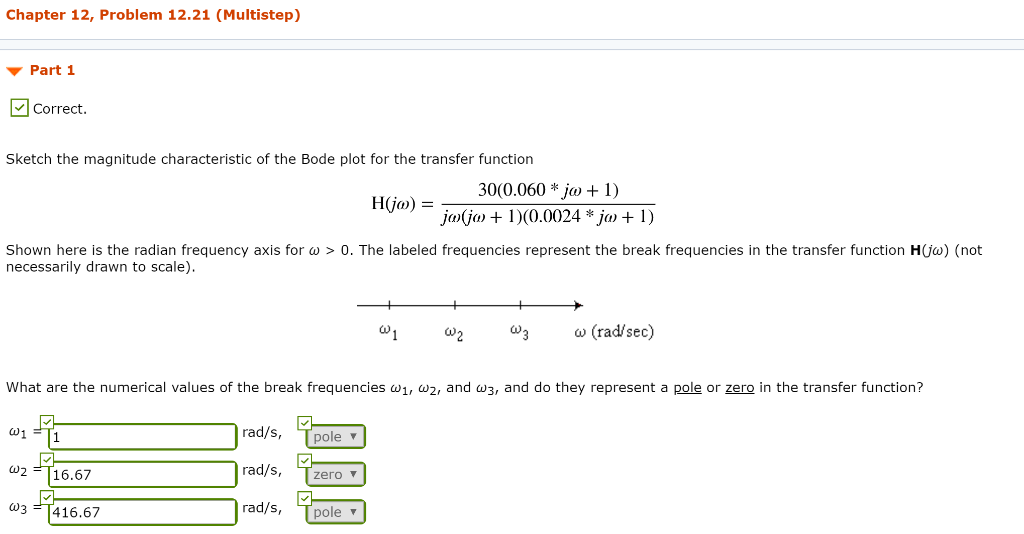Chapter 12, Problem 12.21 (Multistep) Part 1 Correct. Sketch the magnitude characteristic of the Bode plot for the transfer function 30(0.060 * jo + 1) jojo + 1)(0.0024 * ja + 1) H0) = Shown here is the radian frequency axis for w > 0. The labeled frequencies represent the break frequencies in the transfer function H(o) (not necessarily drawn to scale). -+ W + w W3 W (rad/sec) What are the numerical values of the break frequencies w1, wz,...

• ### Chapter 12, Problem 12.16 Describe the sketch of the magnitude characteristic of the Bode plot for...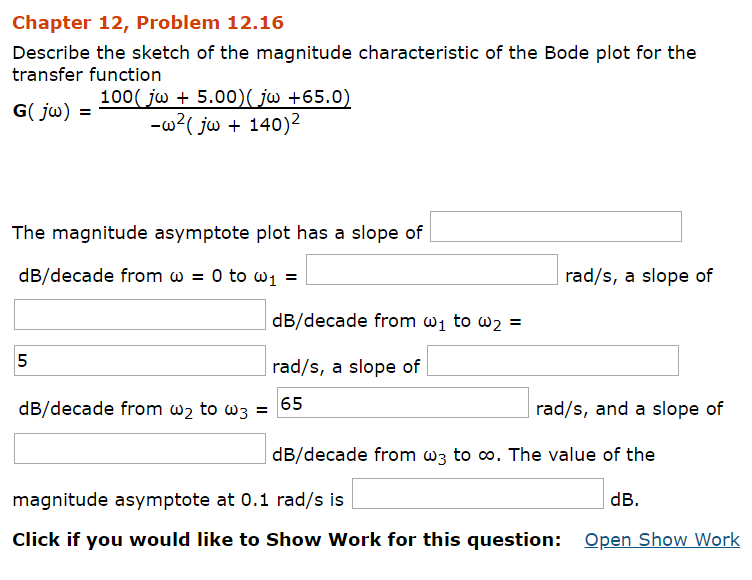Chapter 12, Problem 12.16 Describe the sketch of the magnitude characteristic of the Bode plot for the transfer function The magnitude asymptote plot has a slope of dB/decade from ω-0 to ω! rad/s, a slope of dB/decade from ω1 to ω2- 5 rad/s, a slope of dB/decade from ω2 to ω3-65 rad/s, and a slope of dB/decade from w3 to oo. The value of the magnitude asymptote at 0.1 rad/s is dB. Click if you would like to Show Work...

• ### Sketch the bode plot of a signal conditioner with the transfer function G(s) in the provided...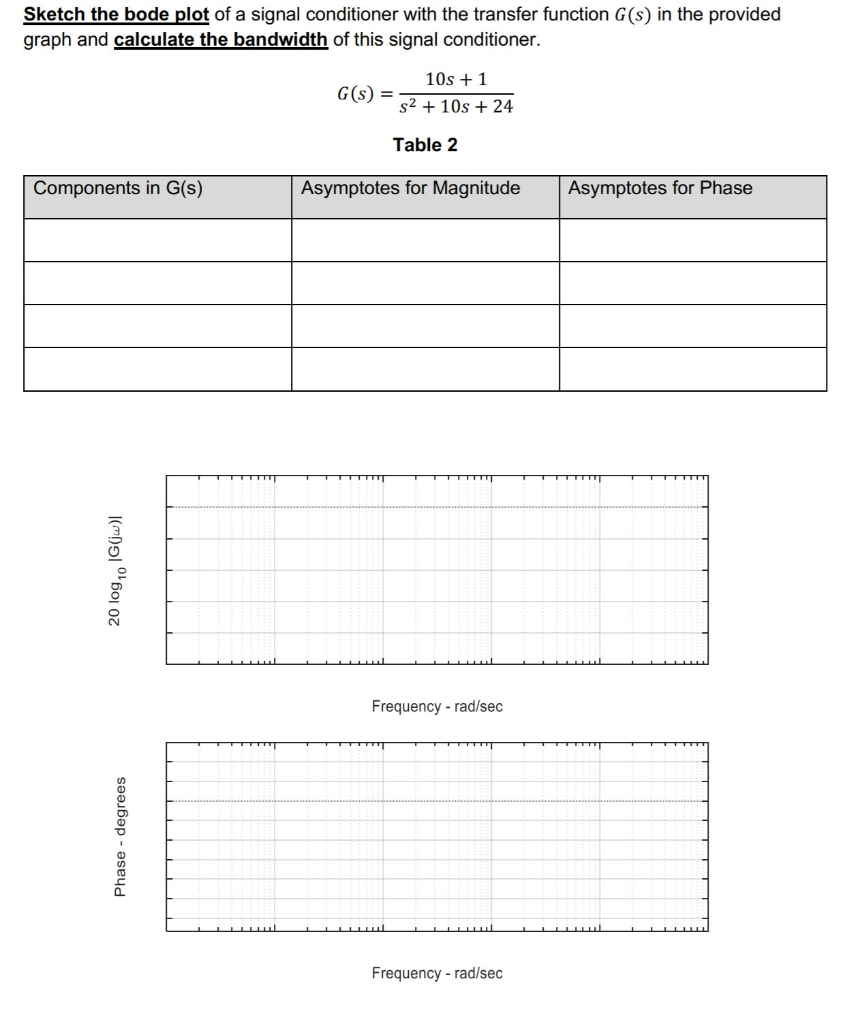Sketch the bode plot of a signal conditioner with the transfer function G(s) in the provided graph and calculate the bandwidth of this signal conditioner. GO 10s +1 S2 + 10s + 24 Table 2 Components in G(S) Asymptotes for Magnitude Asymptotes for Phase 20 log,0 1G(jw) Frequency-rad/sec Phase - degrees Frequency - rad/sec

• ### i) Draw the Bode plots (hand sketch, magnitude and phase!) for the following transfer function. Plot...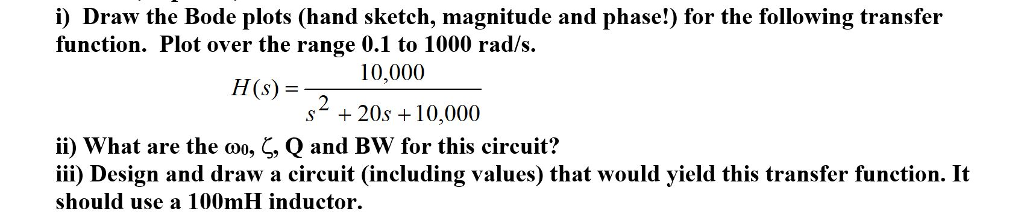i) Draw the Bode plots (hand sketch, magnitude and phase!) for the following transfer function. Plot over the range 0.1 to 1000 rad/s HS 10,000 (s) = s* + 20s 10,000 ii) what are the Q and Bw for this circuit? iii) Design and draw a circuit (including values) that would yield this transfer function. It should use a 100mH inductor , , Qano

• ### 4. Sketch the Bode magnitude plot ofa fiter having a transfer function 10's H(s)- (s+500) (s+S0s+...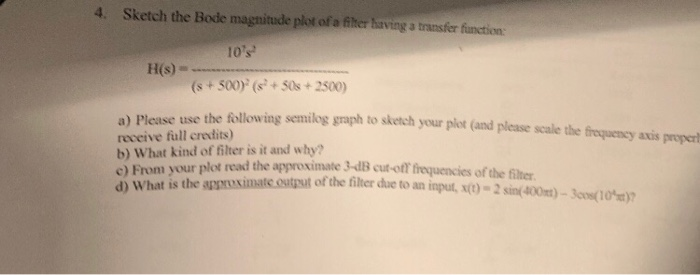4. Sketch the Bode magnitude plot ofa fiter having a transfer function 10's H(s)- (s+500) (s+S0s+2500) he following senilog graph to sketch yo ur plot (and please scale the eequeney axis proper a) Please use t receive full credits) b) What kind of filter is it and why? read the approximate 3-dB cut-off frequencies of the filter From your plot ont is the asrsximate.ostt of the filter due to an input, st)- 2sin(400)-3os(10 a) c) frequency axis properly a) Please...

• ### QUESTION 2 Consider this 2" order transfer function which was discussed in lecture G(s) 10s+9 The Bode plots (magnitude, phase) for this G(s) are provided in this handout. For the following...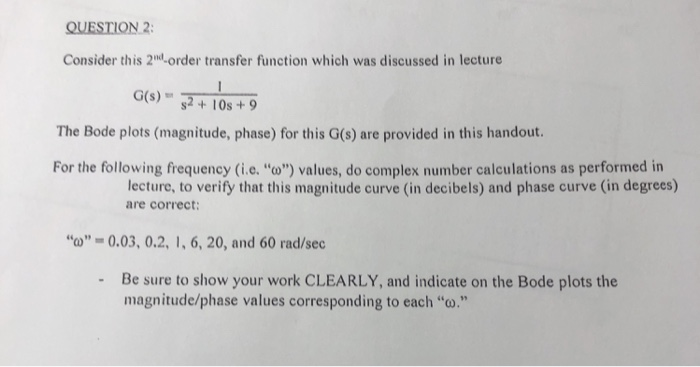QUESTION 2 Consider this 2" order transfer function which was discussed in lecture G(s) 10s+9 The Bode plots (magnitude, phase) for this G(s) are provided in this handout. For the following frequency (i.e."o") values, do complex number calculations as performed in lecture, to verify that this magnitude curve (in decibels) and phase curve (in degrees) are correct “o',-0.03, 0.2, 1, 6, 20, and 60 rad/sec Be sure to show your work CLEARLY, and indicate on the Bode plots the magnitude/phase...

• ### -... Find the transfer function H(w) with the Bode magnitude plot shown in Fig. 14.74 H...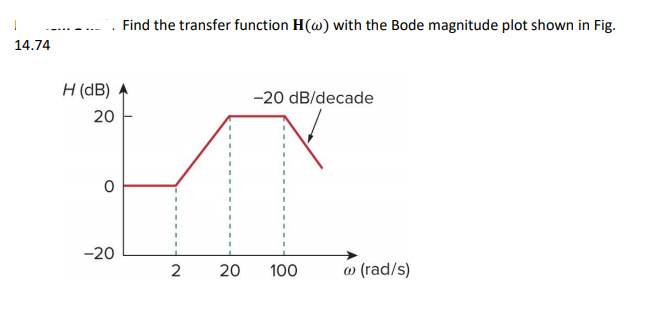-... Find the transfer function H(w) with the Bode magnitude plot shown in Fig. 14.74 H (dB)参 20 20 dB/decade 0 -20L 2 20 100 a) (rad/s)